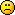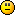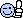Math Is Fun Forum

Discussion about math, puzzles, games and fun.   Useful symbols: ÷ × ½ √ ∞ ≠ ≤ ≥ ≈ ⇒ ± ∈ Δ θ ∴ ∑ ∫  π  -¹ ² ³ °

You are not logged in.

#1 Re: Help Me ! » Need help with Integration of a Cos function » 2009-06-21 03:45:10

Where's the question from?

#2 Re: Help Me ! » Normals » 2009-06-20 05:51:27

I still value the time and effort you spent trying to helpI'm not really sure what you mean. What does "derivation" mean in this context? What do each of the letters represent? Is j the imaginary unit? Is w complex or purely real? Do you want to find a and b in terms of the other letters?

#4 Re: Help Me ! » Normals » 2009-06-19 03:55:49#5 Re: Help Me ! » Normals » 2009-06-18 20:41:28

Ah. Substituting this back into the cubic and squaring gives

This isn't a parabola though. Maybe I got it wrong first time round.

#6 Re: Help Me ! » Normals » 2009-06-18 20:15:33

Okay, thanks for your effort. Thoughts so far below:

For the normal to C at some point (at^2,2at) to pass through a point (h,k), h and k must satisfy

. For exactly two normals to be drawn to the curve and pass through (h,k), there must be exactly two different values of t that satisfy this equation. For a cubic to have exactly two distinct roots, it must have a repeated root.

If we differentiate and set the derivative equal to 0,

. This equation gives the value of t corresponding to the two turning points on the curve, one of which is our double root. Solving for t gives
. In other words, when t is either of these values, the cubic we're interested in has exactly two real roots.

#7 Re: Help Me ! » Normals » 2009-06-18 07:37:03

Thanks. Yes, you're right. I think the question assumes the real numbers though. I wasn't aware cubics had discriminants, either.

You haven't really answered my question though; I'm having trouble finding the Cartesian equation of the locus. When I did this question originally (a few months ago), I remember getting the result that the locus was another parabola (which I thought was rather nice). I can't seem to do it again this time round thoughNo one?

#9 Help Me ! » Normals » 2009-06-17 08:15:37

Daniel123
Replies: 11

Hey, first post for a while.

I did this question a while ago, but I've lost my solution and I can't seem to reproduce it__________________________________________________________________________

Show that the equation

has exactly one real solution if
. [this I can do: differentiating shows the curve is strictly increasing for non-negative p]

A parabola C is given parametrically by

where a is a positive constant.

Find an equation which must be satisfied by t at points on C at which the normal passes through the point (h,k). Hence show that, if

, exactly one normal to C will pass through (h,k) [this I can also do: the equation of the normal to C is
, and we can just sub in (h,k) to find the equation]

Find, in Cartesian form, the equation of the locus of the points from which exactly two normals can be drawn to C.

___________________________________________________________________________

I can't do the last bit this time round. I can see that the equation that must be satisfied by h and k needs to have a repeated root, but that's as far as I can get.

Thanks

#10 Re: Dark Discussions at Cafe Infinity » De- » 2009-05-13 10:25:56

I used to be a cardiologist, but I became disheartened.

#11 Re: Dark Discussions at Cafe Infinity » De- » 2009-05-13 10:21:39

I used to be a fire place, but I was dismantled.

#12 Re: Dark Discussions at Cafe Infinity » De- » 2009-05-13 10:14:50

I used to be a sailor, but I was deported.

#13 Re: Dark Discussions at Cafe Infinity » De- » 2009-05-13 10:04:10

I used to be a pirate, but I was dispatched.

#14 Re: Dark Discussions at Cafe Infinity » De- » 2009-05-13 10:02:16

MathsIsFun wrote:

"I used to be an Electrician, but then I was de-lighted"

Perhaps "discharged" would work better?

Jane's are great#16 Re: Euler Avenue » Nilpotent groups » 2009-05-10 03:44:40

I've been reading it all, in anticipation of learning group theory before I start university (I plan to start learning as soon as my A levels are over).

#17 Re: Help Me ! » Infinites Series » 2009-04-20 07:26:07

I really like it too#18 Re: Help Me ! » Infinites Series » 2009-04-20 07:01:41

Then you can just multiply the LHS by 4 at the end. This is a standard method for series of this type.

#19 Re: Exercises » Snow Ploughs » 2009-04-13 09:02:51#20 Exercises » Snow Ploughs » 2009-04-12 01:31:31

Daniel123
Replies: 3

A lovely problem, taken from Advanced Problems in Mathematics, by Dr Siklos*

Two identical snowploughs plough the same stretch of road. The first starts at a time

seconds after it starts snowing, and the second starts from the same point
seconds later, going in the same direction. Snow falls so that the depth of snow increases at a constant rate
. The speed of each snowplough is
, where
is the depth (in metres) of the snow it is ploughing, and
is a constant. Each snowplough clears all the snow. Show that the time
at which the second snowplough has travelled a distance
metres satisfies the equation:
. Hence show that the snowploughs will collide when they have travelled
metres.

*He adds in his discussion that this can be generalised to n identical snowploughs#21 Re: Help Me ! » u-substitution question » 2009-04-11 23:58:12

JaneFairfax wrote:

Do you mean because

which becomes
after the substitution?

#22 Re: Help Me ! » u-substitution question » 2009-04-11 15:25:27

Because the area of sint cost between 0 and pi is 0 (sint cost = 1/2 sin2t)

#23 Re: Help Me ! » Integration » 2009-04-08 06:47:44

You're absolutely right, TheDude.

Thanks a lot.

#24 Help Me ! » Integration » 2009-04-08 02:45:14

Daniel123
Replies: 2

What am I doing wrong here?

Question

Given that f'(x) > 0 for a ≤ x ≤ b, and that g is the inverse of f, prove, by means of making a suitable change of variable:

where

Let

and let

But

Why am I out by a factor of 2?

Thanks